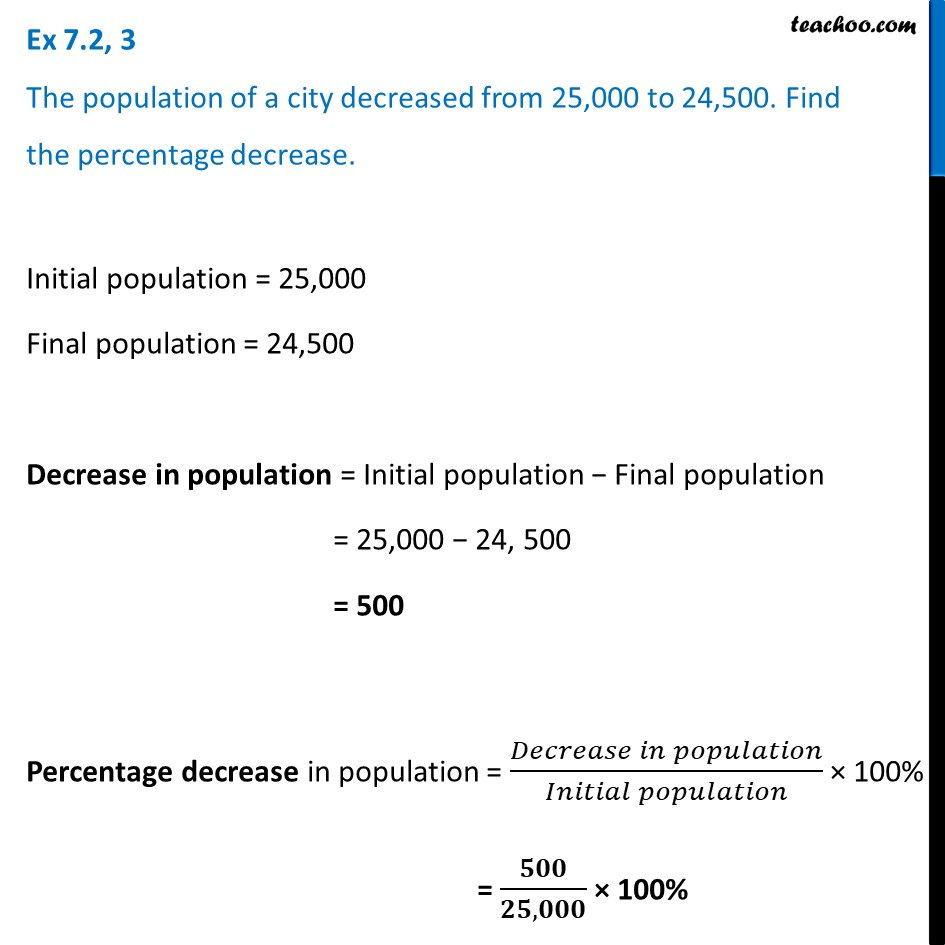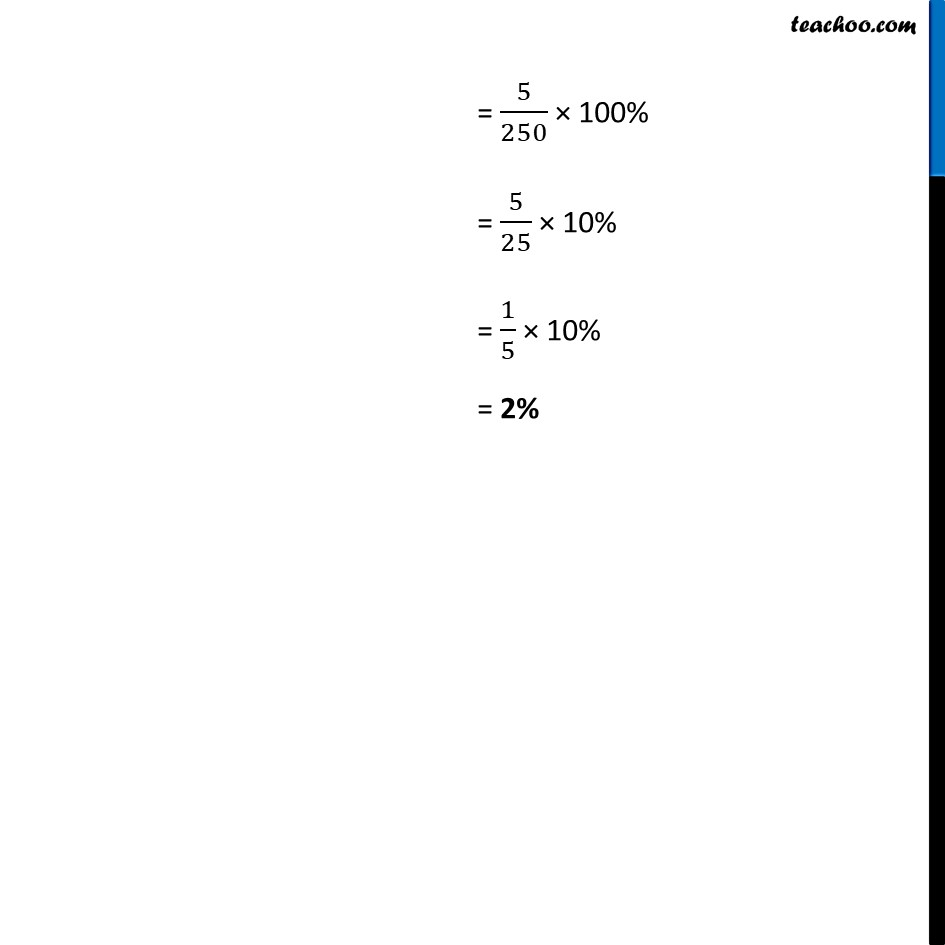Ex 7.2

Chapter 7 Class 7 Comparing Quantities
Serial order wiseLearn in your speed, with individual attention - Teachoo Maths 1-on-1 Class

### Transcript

Ex 7.2, 3 The population of a city decreased from 25,000 to 24,500. Find the percentage decrease. Initial population = 25,000 Final population = 24,500 Decrease in population = Initial population − Final population = 25,000 − 24, 500 = 500 Percentage decrease in population = (𝐷𝑒𝑐𝑟𝑒𝑎𝑠𝑒 𝑖𝑛 𝑝𝑜𝑝𝑢𝑙𝑎𝑡𝑖𝑜𝑛)/(𝐼𝑛𝑖𝑡𝑖𝑎𝑙 𝑝𝑜𝑝𝑢𝑙𝑎𝑡𝑖𝑜𝑛) × 100% = 𝟓𝟎𝟎/(𝟐𝟓,𝟎𝟎𝟎) × 100% = 5/250 × 100% = 5/25 × 10% = 1/5 × 10% = 2%